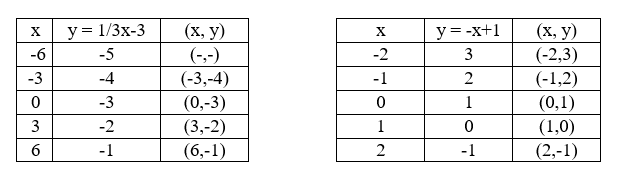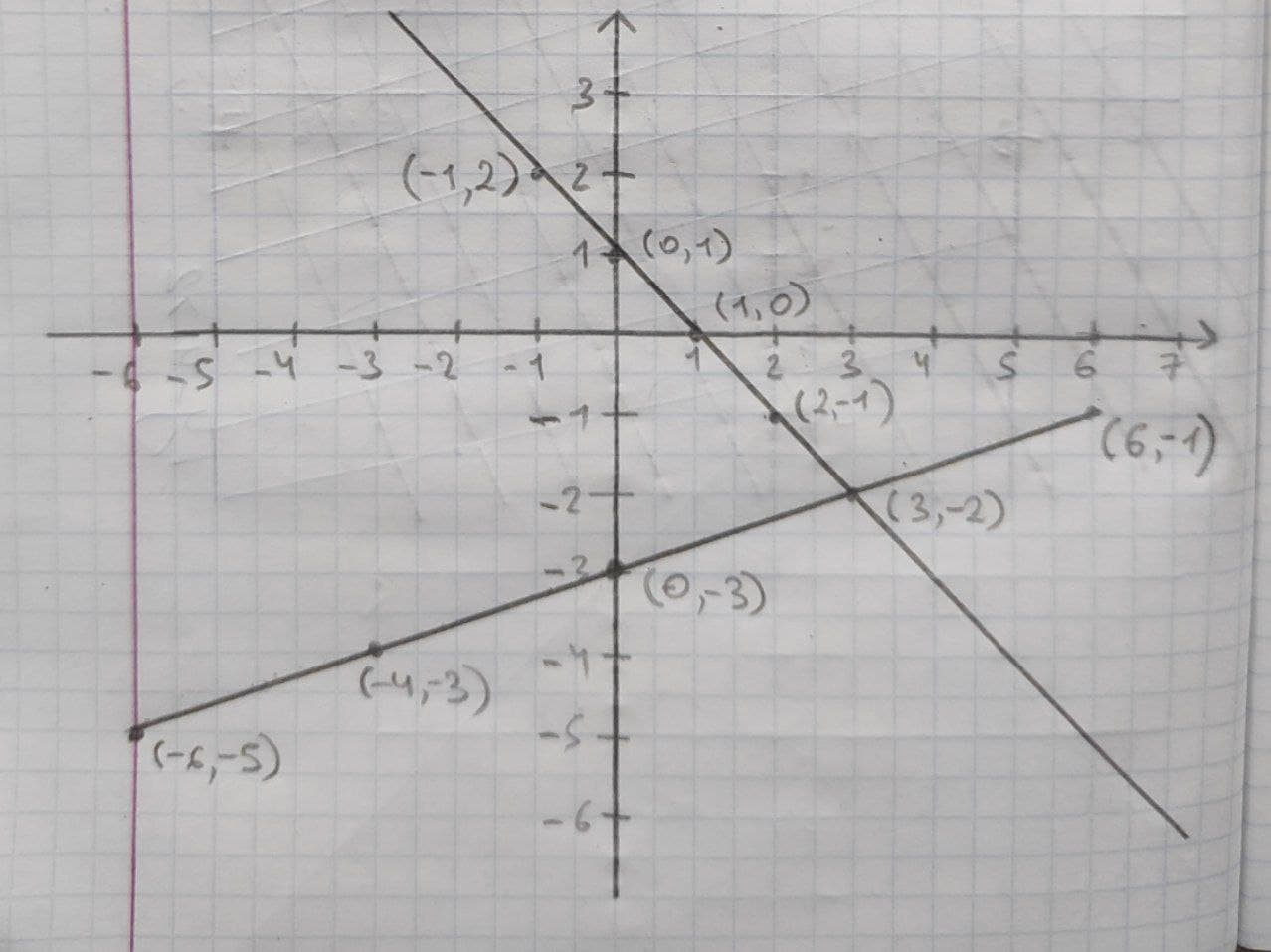# A system of linear equations is a set of two or more equations taken together.melodykap 2020-12-13 Answered

A system of linear equations is a set of two or more equations taken together. The point where the two graphs intersect is called the solution.
$\left\{\begin{array}{c}y=\frac{1}{3}x-3\\ y=-x+1\end{array}$
a) Complete the table fo each linear functionb) Graph both equations on the coordinate plane below

You can still ask an expert for help

• Questions are typically answered in as fast as 30 minutes

Solve your problem for the price of one coffee

• Math expert for every subject
• Pay only if we can solve itNathaniel Kramer
a)The solution of equation= the value of x and y for which both equations satisfy are at x=-2 and y=3
solution=(3,-2)
b)Graph for above table is